Courses

# Chapter 10 Thermodynamic Relations Equilibrium And Stability - Thermodynamics, Mechanical Engineering Mechanical Engineering Notes | EduRev

## Mechanical Engineering : Chapter 10 Thermodynamic Relations Equilibrium And Stability - Thermodynamics, Mechanical Engineering Mechanical Engineering Notes | EduRev

The document Chapter 10 Thermodynamic Relations Equilibrium And Stability - Thermodynamics, Mechanical Engineering Mechanical Engineering Notes | EduRev is a part of the Mechanical Engineering Course Mechanical Engineering SSC JE (Technical).
All you need of Mechanical Engineering at this link: Mechanical Engineering

THERMODYNAMIC RELATIONS EQUILIBRIUM AND STABILITY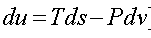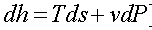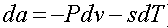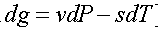where
g is Gibbs function

•  Maxwell's equations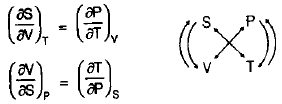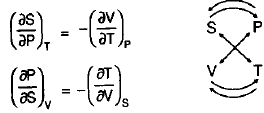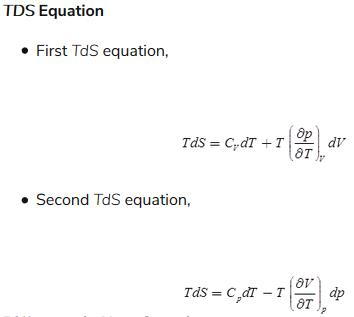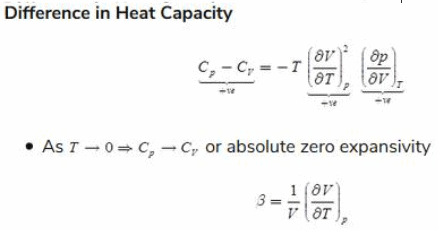Joule-Thompson Effect

When a gas is throttled then first its temperature increase (heating) as the pressure decrease but after a particular pressure, temperature decrease (cooling) as pressure decrease. At different initial temperature different such pressure exist.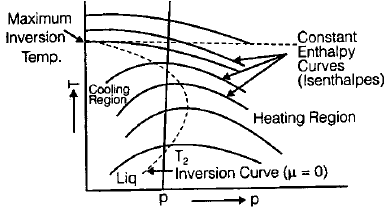•  The curve connecting all transition point is inversion curve.
• The Joule-Thompson Coefficient is: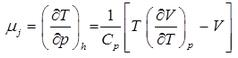• For ideal gas μj = 0 i.e. in throttling process temperature of ideal gas remains constant.
• If initial temperature and pressure are within inversion curve, i.e. below maximum inversion temperature, cooling happens.
• Except Hydrogen and Helium the maximum inversion temperature of all the other gases is more than atmospheric temperature so cooling occurs in throttling of those gases.
• For Hydrogen and Helium maximum inversion temperature is below atmospheric temperature so heating occurs after throttling.
• For cooling of Hydrogen and Helium after throttling, they should initially be cooled below their maximum inversion temperature.
• There is no change in Temperature when an ideal gas is made to under go a Joule-Thompson expansion

Clausius-Clapeyron equation

• Clausis-Clayperon equation is a way of characterizing a discontinuous phase transition between two phases of matter of a single constituent.
• On a P-T diagram, the line separating two phases is known as the coexistence curve.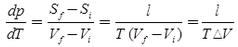where dp/dT is the slope of the tangent to the co-existence curve at any point, l is the specific latent heat, T is the temperature and V is the specific volume change and S stands for specific entropy.

where,

Sf = entropy of the final phase

Si = entropy of the initial phase

Vf = volume of the final phase

Vi = volume of the initial phase

Triple Point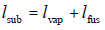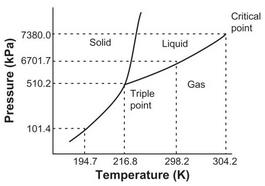Phase diagram for water and any other substance on p–T coordinates.

•  Slope of sublimation curve at the triple point is greater than that of the vaporization curve.

i.e. (dy/dx)sublimation > (dy/dx)vaporization

• Gibbs phase rule for non reactive system

Degree of freedom:

f = c – p + 2
c — no. of components
p — no. of phases

Offer running on EduRev: Apply code STAYHOME200 to get INR 200 off on our premium plan EduRev Infinity!

83 docs|53 tests

,

,

,

,

,

,

,

,

,

,

,

,

,

,

,

,

,

,

,

,

,

,

,

,

;